# Latent Semantic Indexing, SVD, and Zipf’s Law

Latent Semantic Indexing, LSI, uses the Singular Value Decomposition of a term-by-document matrix to represent the information in the documents in a manner that facilitates responding to queries and other information retrieval tasks. I set out to learn for myself how LSI is implemented. I am finding that it is harder than I thought.

### Contents

#### Background

Latent Semantic Indexing was first described in 1990 by Susan Dumais and several colleagues at Bellcore, the descendant of the famous A.T.&T. Bell Labs. (Dumais is now at Microsoft Research.) I first heard about LSI a couple of years later from Mike Berry at the University of Tennessee. Berry, Dumais and Gavin O'Brien have a paper, "Using Linear Algebra for Intelligent Information Retrieval" in SIAM Review in 1995. Here is a link to a preprint of that paper.

An important problem in information retrieval involves synonyms. Different words can refer to the same, or very similar, topics. How can you retrieve a relevant document that does not actually use a key word in a query? For example, consider the terms

• FFT (Finite Fast Fourier Transform)
• SVD (Singular Value Decomposition)
• ODE (Ordinary Differential Equation)

Someone looking for information about PCA (Principal Component Analysis) would be more interested in documents about SVD than those about the other two topics. It is possible to discover this connection because documents about PCA and SVD are more likely to share terms like "rank" and "subspace" that are not present in the others. For information retrieval purposes, PCA and SVD are synonyms. Latent Semantic Indexing can reveal such connections.

#### Strings

I will make use of the new string object, introduced in recent versions of MATLAB. The double quote has been an illegal character in MATLAB. But now it delineates strings.

   s = "% Let $A$ be the 4-by-4 magic square from Durer's _Melancholia_."

s =
"% Let $A$ be the 4-by-4 magic square from Durer's _Melancholia_."


To erase the leading percent sign and the LaTeX between the dollar signs.

   s = erase(s,'%')
s = eraseBetween(s,'$','$','Boundaries','inclusive')

s =
" Let $A$ be the 4-by-4 magic square from Durer's _Melancholia_."
s =
" Let  be the 4-by-4 magic square from Durer's _Melancholia_."


#### Cleve's Corner

The documents for this project are the MATLAB files that constitute the source text for this blog. I started writing the blog edition of Cleve's Corner a little over five years ago, in June 2012.

Each post comes from a MATLAB file processed by the publish command. Most of the lines in the file are comments beginning with a single %. These become the prose in the post. Comments beginning with a double %% generate the title and section headings. Text within '|' characters is rendered with a fixed width font to represent variables and code. Text within '$' characters is LaTeX that is eventually typeset by MathJax. I have collected five years' worth of source files in one directory, Blogs, on my computer. The statement  D = doc_list('Blogs');  produces a string array of the file names in Blogs. The first few lines of D are  D(1:5)  ans = 5×1 string array "apologies_blog.m" "arrowhead2.m" "backslash.m" "balancing.m" "biorhythms.m"  The statements  n = length(D) n/5  n = 135 ans = 27  tell me how many posts I've made, and how many posts per year. That's an average of about one every two weeks. The file that generates today's post is included in the library.  for j = 1:n if contains(D{j},"lsi") j D{j} end end  j = 75 ans = 'lsi_blog.m'  #### Read posts The following code prepends each file name with the directory name, reads each post into a string s, counts the total number of lines that I've written, and computes the average number of lines per post.  lines = 0; for j = 1:n s = read_blog("Blogs/"+D{j}); lines = lines + length(s); end lines avg = lines/n  lines = 15578 avg = 115.3926  #### Stop words The most common word in Cleve's Corner blog is "the". By itself, "the" accounts for over 8 percent of the total number of words. This is roughly the same percentage seen in larger samples of English prose. Stop words are short, frequently used words, like "the", that can be excluded from frequency counts because they are of little value when comparing documents. Here is a list of 25 commonly used stop words. I am going to use a brute force, easy to implement, stategy for stop words. I specify an integer parameter, stop, and simply ignore all words with stop or fewer characters. A value of zero for stop means do not ignore any words. After some experiments which I am about to show, I will decide to take stop = 5. #### Find terms Here is the core code of this project. The input is the list of documents D and the stop parameter stop. The code scans all the documents, finding and counting the individual words. In the process it • skips all noncomment lines • skips all embedded LaTeX • skips all code fragments • skips all URLs • ignores all words with stop or fewer characters The code prints some intermediate results and then returns three arrays. • T, string array of unique words. These are the terms. • C, the frequency counts of T. • A, the (sparse) term/document matrix. The term/document matrix is m -by- n, where • m = length of T is the number of terms, • n = length of D is the number of documents.  type find_terms  function [T,C,A] = find_terms(D,stop) % [T,C,A] = find_terms(D,stop) % Input % D = document list % stop = length of stop words % Output % T = terms, sorted alphabetically % C = counts of terms % A = term/document matrix [W,Cw,wt] = words_and_counts(D,stop); T = terms(W); m = length(T); fprintf('\n\n stop = %d\n words = %d\n m = %d\n\n',stop,wt,m) A = term_doc_matrix(W,Cw,T); C = full(sum(A,2)); [Cp,p] = sort(C,'descend'); Tp = T(p); % Terms sorted by frequency freq = Cp/wt; ten = 10; fprintf(' index term count fraction\n') for k = 1:ten fprintf('%8d %12s %8d %9.4f\n',k,Tp(k),Cp(k),freq(k)) end total = sum(freq(1:ten)); fprintf('%s total = %7.4f\n',blanks(24),total) end  #### No stop words Let's run that code with stop set to zero so there are no stop words. The ten most frequently used words account for over one-quarter of all the words and are useless for characterizing documents. Note that, by itself, "the" accounts for over 8 percent of all the words.  stop = 0; find_terms(D,stop);   stop = 0 words = 114512 m = 8896 index term count fraction 1 the 9404 0.0821 2 of 3941 0.0344 3 a 2885 0.0252 4 and 2685 0.0234 5 is 2561 0.0224 6 to 2358 0.0206 7 in 2272 0.0198 8 that 1308 0.0114 9 for 1128 0.0099 10 this 969 0.0085 total = 0.2577  #### Three characters or less Skipping all words with three or less characters cuts the total number of words almost in half and eliminates "the", but still leaves mostly uninteresting words in the top ten.  stop = 3; find_terms(D,stop);   stop = 3 words = 67310 m = 8333 index term count fraction 1 that 1308 0.0194 2 this 969 0.0144 3 with 907 0.0135 4 matrix 509 0.0076 5 from 481 0.0071 6 matlab 472 0.0070 7 have 438 0.0065 8 about 363 0.0054 9 first 350 0.0052 10 point 303 0.0045 total = 0.0906  #### Five characters or less Setting stop to 4 is a reasonable choice, but let's be even more aggressive. With stop equal to 5, the ten most frequent words are now much more characteristic of this blog. All further calculations use these results.  stop = 5; [T,C,A] = find_terms(D,stop); m = length(T);   stop = 5 words = 38856 m = 6459 index term count fraction 1 matrix 509 0.0131 2 matlab 472 0.0121 3 function 302 0.0078 4 number 224 0.0058 5 floating 199 0.0051 6 precision 184 0.0047 7 computer 167 0.0043 8 algorithm 164 0.0042 9 values 160 0.0041 10 numerical 158 0.0041 total = 0.0653  #### Zipf's Law This is just an aside. Zipf's Law is named after George Kingsley Zipf, although he did not claim originality. The law states that in a sample of natural language, the frequency of any word is inversely proportional to its index in the frequency table. In other words, if the frequency counts C are sorted by frequency, then a plot of log(C(1:k)) versus log(1:k) should be a straight line. Our frequency counts only vaguely follow this law, but a log-log plot makes many quantities look proportional. (We get a little better conformance to the Law with smaller values of stop.)  Zipf(C)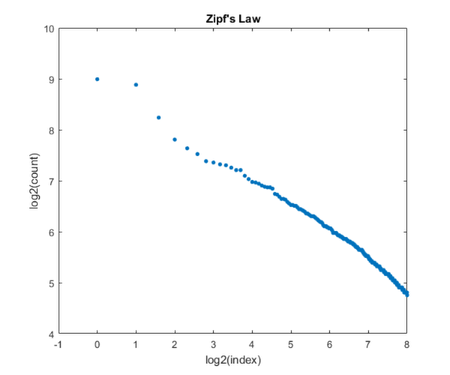#### SVD Now we're ready to compute the SVD. We might as well make A full; it has only n = 135 columns. But A is very tall and skinny, so it's important to use the 'econ' flag so that U also has only 135 columns. Without this flag, we would asking for a square m -by- m matrix U with m over 6000.  [U,S,V] = svd(full(A),'econ');  #### Query Here is a preliminary stab at a function to make queries.  type query   function posts = query(queries,k,cutoff,T,U,S,V,D) % posts = query(queries,k,cutoff,T,U,S,V,D) % Rank k approximation to term/document matrix. Uk = U(:,1:k); Sk = S(1:k,1:k); Vk = V(:,1:k); % Construct the query vector from the relevant terms. m = size(U,1); q = zeros(m,1); for i = 1:length(queries) % Find the index of the query key word in the term list. wi = word_index(queries{i},T); q(wi) = 1; end % Project the query onto the document space. qhat = Sk\Uk'*q; v = Vk*qhat; v = v/norm(qhat); % Pick off the documents that are close to the query. posts = D(v > cutoff); query_plot(v,cutoff,queries) end  #### Try it Set the rank k and the cutoff level.  rank = 40; cutoff = .2;  Try a few one-word queries.  queries = {"singular","roundoff","wilkinson"}; for j = 1:length(queries) queryj = queries{j} posts = query(queryj,rank,cutoff,T,U,S,V,D) snapnow end  queryj = "singular" posts = 4×1 string array "eigshowp_w3.m" "four_spaces_blog.m" "parter_blog.m" "svdshow_blog.m"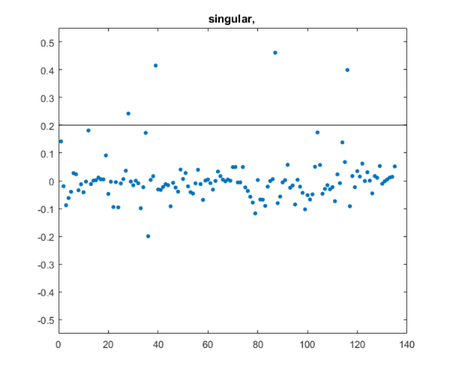queryj = "roundoff" posts = 5×1 string array "condition_blog.m" "floats.m" "partial_pivot_blog.m" "refine_blog.m" "sanderson_blog.m"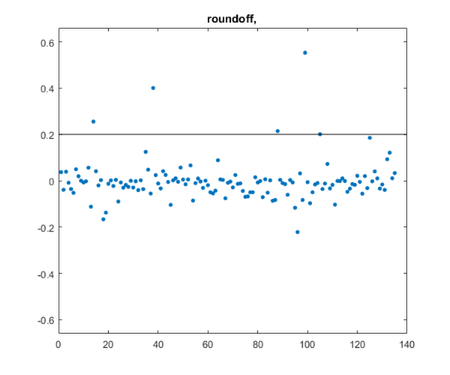queryj = "wilkinson" posts = 2×1 string array "dubrulle.m" "jhw_1.m"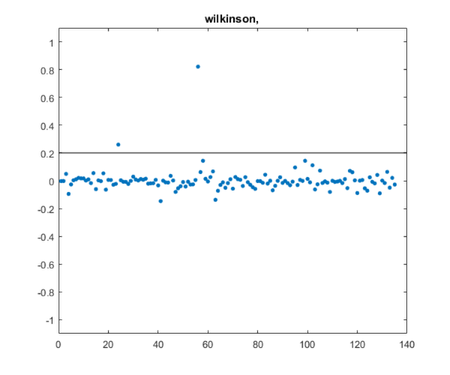Note that the query about Wilkinson found the post about him and also the post about Dubrulle, who improved a Wilkinson algorithm. For the finale, merge all the queries into one.  queries posts = query(queries,rank,cutoff,T,U,S,V,D)  queries = 1×3 cell array ["singular"] ["roundoff"] ["wilkinson"] posts = 5×1 string array "four_spaces_blog.m" "jhw_1.m" "parter_blog.m" "refine_blog.m" "svdshow_blog.m"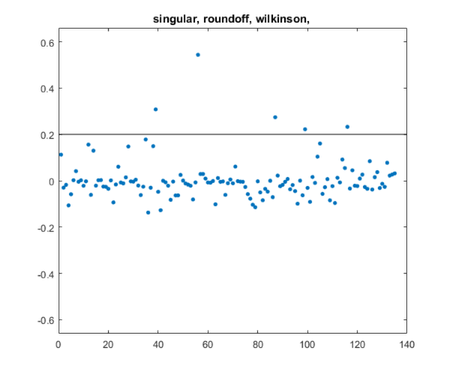------------------------------------------------------------------ #### Code doc_list  type doc_list  function D = doc_list(dir) % D = doc_list(dir) is a string array of the file names in 'dir'. D = ""; [status,L] = system(['ls ' dir]); if status ~= 0 error(['Cannot find ' dir]) end k1 = 1; i = 1; for k2 = find(L == newline) D(i,:) = L(k1:k2-1); i = i+1; k1 = k2+1; end end  erase_stuff  type erase_stuff  function sout = erase_stuff(s) % s = erase_stuff(s) % erases noncomments, !system, LaTeX, URLs, Courier, and punctuation. j = 1; k = 1; while k <= length(s) sk = lower(char(s(k))); if length(sk) >= 4 if sk(1) ~= '%' % Skip non comment break elseif any(sk=='!') && any(sk=='|') % Skip !system | ... break elseif all(sk(3:4) == '$')
sk(3:4) = '  ';
% Skip display latex
while all(sk~='$') k = k+1; sk = char(s(k)); end else % Embedded latex sk = eraseBetween(sk,'$','\$','Boundaries','inclusive');

% URL
if any(sk == '<')
if ~any(sk == '>')
k = k+1;
sk = [sk lower(char(s(k)))];
end
sk = eraseBetween(sk,'<','>','Boundaries','inclusive');
sk = erase(sk,'>');
end
if contains(string(sk),"http")
break
end

% Courier
f = find(sk == '|');
assert(length(f)==1 | mod(length(f),2)==0);
for i = 1:2:length(f)-1
w = sk(f(i)+1:f(i+1)-1);
if length(w)>2 && all(w>='a') && all(w<='z')
sk(f(i)) = ' ';
sk(f(i+1)) = ' ';
else
sk(f(i):f(i+1)) = ' ';
end
end

% Puncuation
sk((sk<'a' | sk>'z') & (sk~=' ')) = [];

sout(j,:) = string(sk);
j = j+1;
end
end
k = k+1;
end
skip = k-j;
end


find_words

   type find_words

function w = find_words(s,stop)
% words(s,stop)   Find the words with length > stop in the text string.
w = "";
i = 1;
for k = 1:length(s)
sk = [' ' char(s{k}) ' '];
f = strfind(sk,' ');
for j = 1:length(f)-1
t = strtrim(sk(f(j):f(j+1)));
% Skip stop words
if length(t) <= stop
continue
end
if ~isempty(t)
w{i,1} = t;
i = i+1;
end
end
end


query_plot

   type query_plot

function query_plot(v,cutoff,queries)
clf
shg
plot(v,'.','markersize',12)
ax = axis;
yax = 1.1*max(abs(ax(3:4)));
axis([ax(1:2) -yax yax])
line(ax(1:2),[cutoff cutoff],'color','k')
title(sprintf('%s, ',queries{:}))
end


   type read_blog

function s = read_blog(filename)
% skip over lines that do not begin with '%'.
fid = fopen(filename);
line = fgetl(fid);
k = 1;
while ~isequal(line,-1)  % -1 signals eof
if length(line) > 2 && line(1) == '%'
s(k,:) = string(line);
k = k+1;
end
line = fgetl(fid);
end
fclose(fid);
end


term_doc_matrix

   type term_doc_matrix

function A = term_doc_matrix(W,C,T)
% A = term_doc_matrix(W,C,T)
m = length(T);
n = length(W);
A = sparse(m,n);
for j = 1:n
nzj = length(W{j});
i = zeros(nzj,1);
s = zeros(nzj,1);
for k = 1:nzj
i(k) = word_index(W{j}(k),T);
s(k) = C{j}(k);
end
A(i,j) = s;
end
end


word_count

   type word_count

function [wout,count] = word_count(w)
% [w,count] = word_count(w)   Unique words with counts.
w = sort(w);
wout = "";
count = [];
i = 1;
k = 1;
while k <= length(w)
c = 1;
while k < length(w) && isequal(w{k},w{k+1})
c = c+1;
k = k+1;
end
wout{i,1} = w{k};
count(i,1) = c;
i = i+1;
k = k+1;
end
[count,p] = sort(count,'descend');
wout = wout(p);
end


word_index

   type word_index

function p = word_index(w,list)
% Index of w in list.
% Returns empty if w is not in list.
m = length(list);
p = fix(m/2);
q = ceil(p/2);
t = 0;
tmax = ceil(log2(m));
% Binary search
while list(p) ~= w
if list(p) > w
p = max(p-q,1);
else
p = min(p+q,m);
end
q = ceil(q/2);
t = t+1;
if t == tmax
p = [];
break
end
end
end


words_and_counts

   type words_and_counts

function [W,Cw,wt] = words_and_counts(D,stop)
% [W,Cw,wt] = words_and_counts(D,stop)
W = [];  % Words
Cw = [];  % Counts
wt = 0;
n = length(D);
for j = 1:n
s = erase_stuff(s);
w = find_words(s,stop);
[W{j,1},Cw{j,1}] = word_count(w);
wt = wt + length(w);
end
end


Zipf

   type Zipf

function Zipf(C)
% Zipf(C) plots log2(C(1:k)) vs. log2(1:k)
C = sort(C,'descend');  % Sort counts by frequency
figure(1)
clf
shg
k = 256;
plot(log2(1:k),log2(C(1:k)),'.','markersize',12)
axis([-1 8 4 10])
xlabel('log2(index)')
ylabel('log2(count)')
title('Zipf''s Law')
drawnow
end


Published with MATLAB® R2017a

|